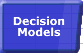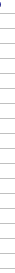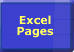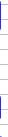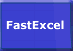# Excel's Smart Recalculation EngineSmart Recalculation Evaluation Circumstances Calculation Process Dependency Trees Controlling Calculation "CALCULATE" in Status Bar Calculation Methods Calculating from VBA Volatile Functions & Actions User-Defined Functions Repetitive Calculation Version Timing Comparison## Excels Smart Recalculation Engine

Excel has a complex and finely tuned algorithm for choosing the fastest sequence and the minimum number of cells required to calculate the correct answer.
This algorithm was changed significantly when the calculation engine was rewritten in Excel 97, and further changes have been made in Excel 2002 and 2007.

 Excel normally only calculates the minimum number of cells possible. Excels smart recalculation engine normally minimises calculation time by tracking changes and only recalculating Cells, formulae, values or names that have changed or are flagged as needing recalculation. Cells dependent on other cells, formulae, names or values that need recalculation. However Defined Names are recalculated each time a formula that directly refers to the name is recalculated. Note that the process of calculating cells that are dependent on previously calculated cells continues even if the value of a cell does not change when it is calculated. This smart recalculation usually only takes a fraction of the time for a full calculation of all the formulae in the workbook, although sometimes the extra work required can make it slower than a full calculation. So if a recalculation takes a long time, try a full calculation to see if its faster.

Worksheets and Workbooks are then also flagged as containing uncalculated cells.

There are exceptions to this change tracking and smart recalculation process:

• From Excel 2000 opening a workbook in Automatic mode that was last calculated (and saved) in an earlier version (which includes earlier versions of the calculation engine) gives you a message saying "Microsoft Excel recalculates formulas when opening files last saved by an earlier version of Excel". This also causes a Full Calculation rather than a smart recalculation.
Jim Rech has posted a registry fix that prevents the Full calculation:
- To stop this you need to make a registry entry (Start->Run "Regedit" (no quotes)).
- Under: HKEY_CURRENT_USER\Software\Microsoft\Office\9.0\Excel\Options create a new DWORD item named: FullCalcOnLoadOldFile
- Leave its value at the default of 0.
• Volatile functions are always calculated.
• Full Calculation (Ctrl-Alt-F9), which calculates ALL cells.
• Setting the Workbook.ForceFullCalculation property to True will make each calculation a Full calculation and will not update the dependency trees.
• More than 65536 Dependencies , which causes full calculations rather than smart recalculations.
• Setting the Worksheet.EnableCalculation property to False will prevent uncalculated cells on a worksheet from being calculated. Setting Worksheet.EnableCalculation back to True will then cause all the formulae on the sheet to be flagged as uncalculated.
• Unused names are never calculated.
• Names are calculated each time they are referenced by a formula that is recalculated.© 2001-2020 Decision Models Legal Disclaimer Privacy Statement